# Confidence Level and Sample Size

7 de Feb de 2019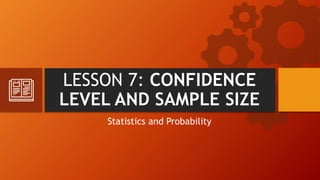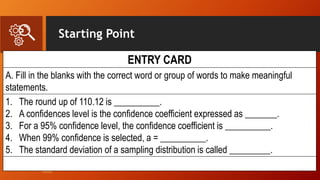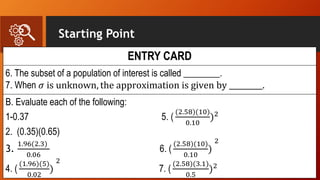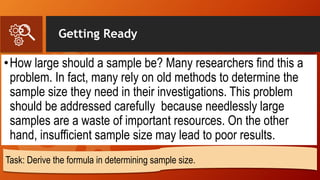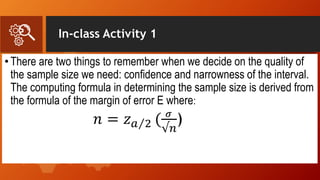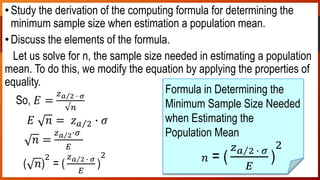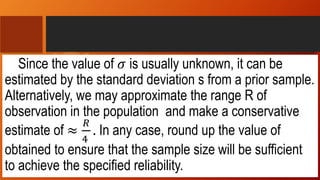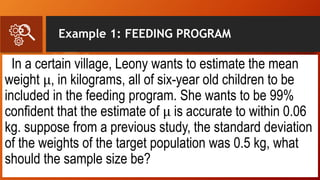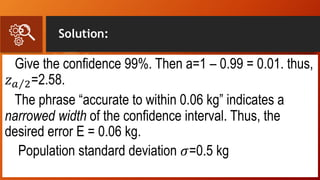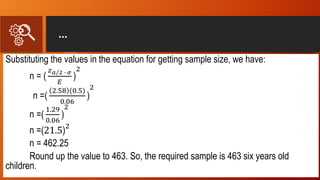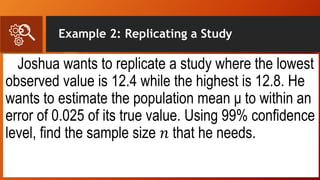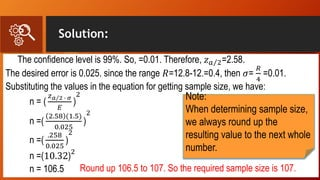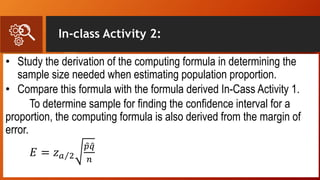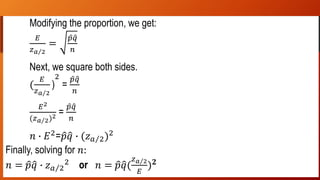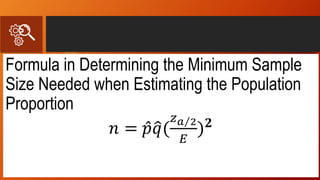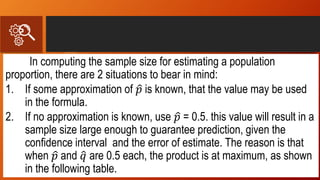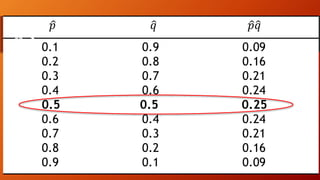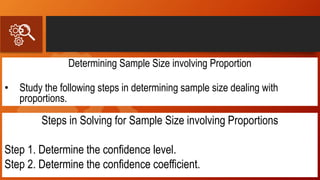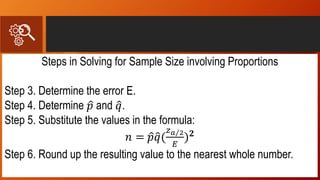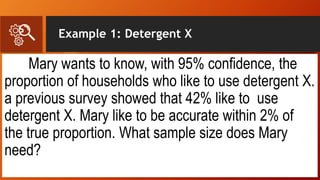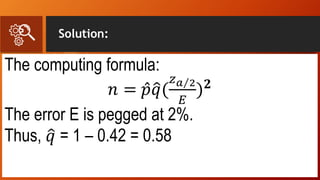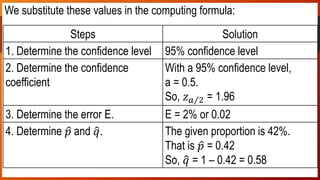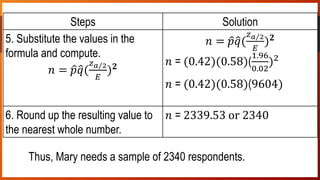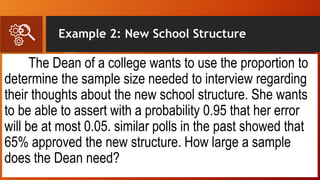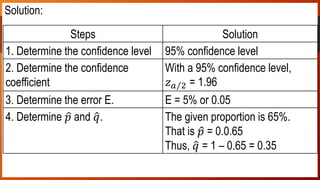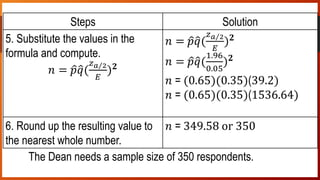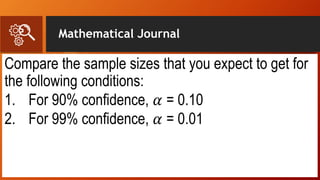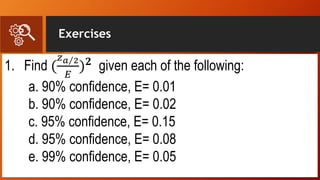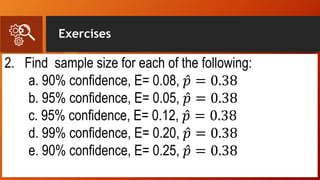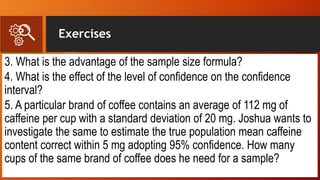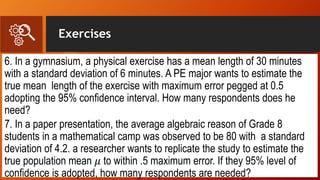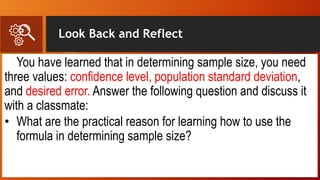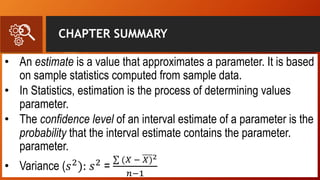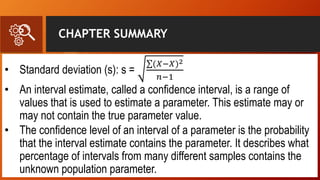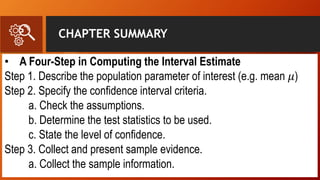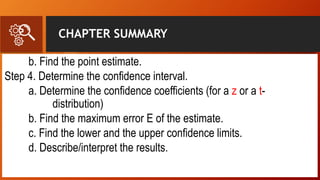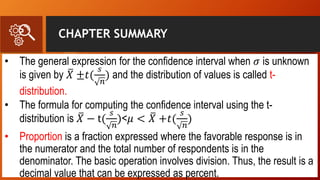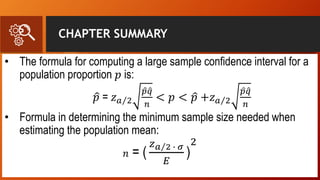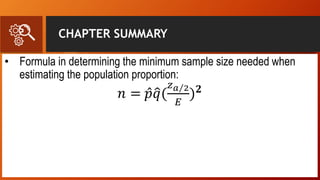1 de 40

### Confidence Level and Sample Size

• 1. LESSON 7: CONFIDENCE LEVEL AND SAMPLE SIZE Statistics and Probability
• 2. Starting Point ENTRY CARD A. Fill in the blanks with the correct word or group of words to make meaningful statements. 1. The round up of 110.12 is __________. 2. A confidences level is the confidence coefficient expressed as _______. 3. For a 95% confidence level, the confidence coefficient is __________. 4. When 99% confidence is selected, a = __________. 5. The standard deviation of a sampling distribution is called _________.
• 3. Starting Point ENTRY CARD 6. The subset of a population of interest is called ________. 7. When 𝜎 is unknown, the approximation is given by _________. B. Evaluate each of the following: 1-0.37 5. ( (2.58)(10) 0.10 )2 2. (0.35)(0.65) 3. 1.96(2.3) 0.06 6. ( (2.58)(10) 0.10 ) 2 4. ( (1.96)(5) 0.02 ) 2 7. ( (2.58)(3.1) 0.5 )2
• 4. Getting Ready •How large should a sample be? Many researchers find this a problem. In fact, many rely on old methods to determine the sample size they need in their investigations. This problem should be addressed carefully because needlessly large samples are a waste of important resources. On the other hand, insufficient sample size may lead to poor results. Task: Derive the formula in determining sample size.
• 5. In-class Activity 1 •There are two things to remember when we decide on the quality of the sample size we need: confidence and narrowness of the interval. The computing formula in determining the sample size is derived from the formula of the margin of error E where: 𝑛 = 𝑧 𝑎 2 ( 𝜎 √𝑛 )
• 6. In-class Activity 1 •Study the derivation of the computing formula for determining the minimum sample size when estimation a population mean. •Discuss the elements of the formula. Let us solve for n, the sample size needed in estimating a population mean. To do this, we modify the equation by applying the properties of equality. So, 𝐸 = 𝑧 𝑎 2 ∙ 𝜎 √𝑛 𝐸 𝑛 = 𝑧 𝑎 2 ∙ 𝜎 𝑛 = 𝑧 𝑎 2∙𝜎 𝐸 ( 𝑛) 2 = ( 𝑧 𝑎 2 ∙ 𝜎 𝐸 ) 2 Formula in Determining the Minimum Sample Size Needed when Estimating the Population Mean 𝑛 = ( 𝑧 𝑎 2 ∙ 𝜎 𝐸 ) 2
• 7. Since the value of 𝜎 is usually unknown, it can be estimated by the standard deviation s from a prior sample. Alternatively, we may approximate the range R of observation in the population and make a conservative estimate of ≈ 𝑅 4 . In any case, round up the value of obtained to ensure that the sample size will be sufficient to achieve the specified reliability.
• 8. Example 1: FEEDING PROGRAM In a certain village, Leony wants to estimate the mean weight μ, in kilograms, all of six-year old children to be included in the feeding program. She wants to be 99% confident that the estimate of μ is accurate to within 0.06 kg. suppose from a previous study, the standard deviation of the weights of the target population was 0.5 kg, what should the sample size be?
• 9. Solution: Give the confidence 99%. Then a=1 – 0.99 = 0.01. thus, 𝑧 𝑎 2=2.58. The phrase “accurate to within 0.06 kg” indicates a narrowed width of the confidence interval. Thus, the desired error E = 0.06 kg. Population standard deviation 𝜎=0.5 kg
• 10. … Substituting the values in the equation for getting sample size, we have: n = ( 𝑧 𝑎 2 ∙ 𝜎 𝐸 ) 2 n =( 2.58 (0.5) 0.06 ) 2 n =( 1.29 0.06 ) 2 n =(21.5) 2 n = 462.25 Round up the value to 463. So, the required sample is 463 six years old children.
• 11. Example 2: Replicating a Study Joshua wants to replicate a study where the lowest observed value is 12.4 while the highest is 12.8. He wants to estimate the population mean μ to within an error of 0.025 of its true value. Using 99% confidence level, find the sample size 𝑛 that he needs.
• 12. Solution: The confidence level is 99%. So, =0.01. Therefore, 𝑧 𝑎 2=2.58. The desired error is 0.025. since the range 𝑅=12.8-12.=0.4, then 𝜎= 𝑅 4 =0.01. Substituting the values in the equation for getting sample size, we have: n = ( 𝑧 𝑎 2 ∙ 𝜎 𝐸 ) 2 n =( 2.58 (1.5) 0.025 ) 2 n =( .258 0.025 ) 2 n =(10.32) 2 n = 106.5 Round up 106.5 to 107. So the required sample size is 107. Note: When determining sample size, we always round up the resulting value to the next whole number.
• 13. In-class Activity 2: • Study the derivation of the computing formula in determining the sample size needed when estimating population proportion. • Compare this formula with the formula derived In-Cass Activity 1. To determine sample for finding the confidence interval for a proportion, the computing formula is also derived from the margin of error. 𝐸 = 𝑧 𝑎 2 𝑝 𝑞 𝑛
• 14. … Modifying the proportion, we get: 𝐸 𝑧 𝑎 2 = 𝑝 𝑞 𝑛 Next, we square both sides. ( 𝐸 𝑧 𝑎 2 ) 2 = 𝑝 𝑞 𝑛 𝐸2 (𝑧 𝑎 2)2 = 𝑝 𝑞 𝑛 𝑛 ∙ 𝐸2 = 𝑝 𝑞 ∙ (𝑧 𝑎 2)2 Finally, solving for 𝑛: 𝑛 = 𝑝 𝑞 ∙ 𝑧 𝑎 2 2 or 𝑛 = 𝑝 𝑞( 𝑧 𝑎 2 𝐸 ) 𝟐
• 15. Formula in Determining the Minimum Sample Size Needed when Estimating the Population Proportion 𝑛 = 𝑝 𝑞( 𝑧 𝑎 2 𝐸 ) 𝟐
• 16. In computing the sample size for estimating a population proportion, there are 2 situations to bear in mind: 1. If some approximation of 𝑝 is known, that the value may be used in the formula. 2. If no approximation is known, use 𝑝 = 0.5. this value will result in a sample size large enough to guarantee prediction, given the confidence interval and the error of estimate. The reason is that when 𝑝 and 𝑞 are 0.5 each, the product is at maximum, as shown in the following table.
• 17. 𝑝 𝑞 𝑝 𝑞 0.1 0.9 0.09 0.2 0.8 0.16 0.3 0.7 0.21 0.4 0.6 0.24 0.5 0.5 0.25 0.6 0.4 0.24 0.7 0.3 0.21 0.8 0.2 0.16 0.9 0.1 0.09
• 18. Determining Sample Size involving Proportion • Study the following steps in determining sample size dealing with proportions. Steps in Solving for Sample Size involving Proportions Step 1. Determine the confidence level. Step 2. Determine the confidence coefficient.
• 19. Steps in Solving for Sample Size involving Proportions Step 3. Determine the error E. Step 4. Determine 𝑝 and 𝑞. Step 5. Substitute the values in the formula: 𝑛 = 𝑝 𝑞( 𝑧 𝑎 2 𝐸 ) 𝟐 Step 6. Round up the resulting value to the nearest whole number.
• 20. Example 1: Detergent X Mary wants to know, with 95% confidence, the proportion of households who like to use detergent X. a previous survey showed that 42% like to use detergent X. Mary like to be accurate within 2% of the true proportion. What sample size does Mary need?
• 21. Solution: The computing formula: 𝑛 = 𝑝 𝑞( 𝑧 𝑎 2 𝐸 ) 𝟐 The error E is pegged at 2%. Thus, 𝑞 = 1 – 0.42 = 0.58
• 22. Solution: We substitute these values in the computing formula: Steps Solution 1. Determine the confidence level 95% confidence level 2. Determine the confidence coefficient With a 95% confidence level, a = 0.5. So, 𝑧 𝑎 2 = 1.96 3. Determine the error E. E = 2% or 0.02 4. Determine 𝑝 and 𝑞. The given proportion is 42%. That is 𝑝 = 0.42 So, 𝑞 = 1 – 0.42 = 0.58
• 23. Solution: Thus, Mary needs a sample of 2340 respondents. Steps Solution 5. Substitute the values in the formula and compute. 𝑛 = 𝑝 𝑞( 𝑧 𝑎 2 𝐸 ) 𝟐 𝑛 = 𝑝 𝑞( 𝑧 𝑎 2 𝐸 ) 𝟐 𝑛 = (0.42)(0.58)( 1.96 0.02 )2 𝑛 = (0.42)(0.58)(9604) 6. Round up the resulting value to the nearest whole number. 𝑛 = 2339.53 or 2340
• 24. Example 2: New School Structure The Dean of a college wants to use the proportion to determine the sample size needed to interview regarding their thoughts about the new school structure. She wants to be able to assert with a probability 0.95 that her error will be at most 0.05. similar polls in the past showed that 65% approved the new structure. How large a sample does the Dean need?
• 25. Solution: Solution: Steps Solution 1. Determine the confidence level 95% confidence level 2. Determine the confidence coefficient With a 95% confidence level, 𝑧 𝑎 2 = 1.96 3. Determine the error E. E = 5% or 0.05 4. Determine 𝑝 and 𝑞. The given proportion is 65%. That is 𝑝 = 0.0.65 Thus, 𝑞 = 1 – 0.65 = 0.35
• 26. Solution: The Dean needs a sample size of 350 respondents. Steps Solution 5. Substitute the values in the formula and compute. 𝑛 = 𝑝 𝑞( 𝑧 𝑎 2 𝐸 ) 𝟐 𝑛 = 𝑝 𝑞( 𝑧 𝑎 2 𝐸 ) 𝟐 𝑛 = 𝑝 𝑞( 1.96 0.05 ) 𝟐 𝑛 = (0.65)(0.35)(39.2) 𝑛 = (0.65)(0.35)(1536.64) 6. Round up the resulting value to the nearest whole number. 𝑛 = 349.58 or 350
• 27. Mathematical Journal Compare the sample sizes that you expect to get for the following conditions: 1. For 90% confidence, 𝛼 = 0.10 2. For 99% confidence, 𝛼 = 0.01
• 28. Exercises 1. Find ( 𝑧 𝑎 2 𝐸 ) 𝟐 given each of the following: a. 90% confidence, E= 0.01 b. 90% confidence, E= 0.02 c. 95% confidence, E= 0.15 d. 95% confidence, E= 0.08 e. 99% confidence, E= 0.05
• 29. Exercises 2. Find sample size for each of the following: a. 90% confidence, E= 0.08, 𝑝 = 0.38 b. 95% confidence, E= 0.05, 𝑝 = 0.38 c. 95% confidence, E= 0.12, 𝑝 = 0.38 d. 99% confidence, E= 0.20, 𝑝 = 0.38 e. 90% confidence, E= 0.25, 𝑝 = 0.38
• 30. Exercises 3. What is the advantage of the sample size formula? 4. What is the effect of the level of confidence on the confidence interval? 5. A particular brand of coffee contains an average of 112 mg of caffeine per cup with a standard deviation of 20 mg. Joshua wants to investigate the same to estimate the true population mean caffeine content correct within 5 mg adopting 95% confidence. How many cups of the same brand of coffee does he need for a sample?
• 31. Exercises 6. In a gymnasium, a physical exercise has a mean length of 30 minutes with a standard deviation of 6 minutes. A PE major wants to estimate the true mean length of the exercise with maximum error pegged at 0.5 adopting the 95% confidence interval. How many respondents does he need? 7. In a paper presentation, the average algebraic reason of Grade 8 students in a mathematical camp was observed to be 80 with a standard deviation of 4.2. a researcher wants to replicate the study to estimate the true population mean 𝜇 to within .5 maximum error. If they 95% level of confidence is adopted, how many respondents are needed?
• 32. Look Back and Reflect You have learned that in determining sample size, you need three values: confidence level, population standard deviation, and desired error. Answer the following question and discuss it with a classmate: • What are the practical reason for learning how to use the formula in determining sample size?
• 33. CHAPTER SUMMARY • An estimate is a value that approximates a parameter. It is based on sample statistics computed from sample data. • In Statistics, estimation is the process of determining values parameter. • The confidence level of an interval estimate of a parameter is the probability that the interval estimate contains the parameter. parameter. • Variance (𝑠2 ): 𝑠2 = (𝑋 − 𝑋)2 𝑛−1
• 34. CHAPTER SUMMARY • Standard deviation (s): s = (𝑋−𝑋)2 𝑛−1 • An interval estimate, called a confidence interval, is a range of values that is used to estimate a parameter. This estimate may or may not contain the true parameter value. • The confidence level of an interval of a parameter is the probability that the interval estimate contains the parameter. It describes what percentage of intervals from many different samples contains the unknown population parameter.
• 35. CHAPTER SUMMARY • A Four-Step in Computing the Interval Estimate Step 1. Describe the population parameter of interest (e.g. mean 𝜇) Step 2. Specify the confidence interval criteria. a. Check the assumptions. b. Determine the test statistics to be used. c. State the level of confidence. Step 3. Collect and present sample evidence. a. Collect the sample information.
• 36. CHAPTER SUMMARY b. Find the point estimate. Step 4. Determine the confidence interval. a. Determine the confidence coefficients (for a z or a t- distribution) b. Find the maximum error E of the estimate. c. Find the lower and the upper confidence limits. d. Describe/interpret the results.
• 37. CHAPTER SUMMARY • The general expression for the confidence interval when 𝜎 is unknown is given by 𝑋 ±𝑡( 𝑠 𝑛 ) and the distribution of values is called t- distribution. • The formula for computing the confidence interval using the t- distribution is 𝑋 − t( 𝑠 𝑛 )<𝜇 < 𝑋 +𝑡( 𝑠 𝑛 ) • Proportion is a fraction expressed where the favorable response is in the numerator and the total number of respondents is in the denominator. The basic operation involves division. Thus, the result is a decimal value that can be expressed as percent.
• 38. CHAPTER SUMMARY • The formula for computing a large sample confidence interval for a population proportion 𝑝 is: 𝑝 = 𝑧 𝑎 2 𝑝 𝑞 𝑛 < 𝑝 < 𝑝 +𝑧 𝑎 2 𝑝 𝑞 𝑛 • Formula in determining the minimum sample size needed when estimating the population mean: 𝑛 = ( 𝑧 𝑎 2 ∙ 𝜎 𝐸 ) 2
• 39. CHAPTER SUMMARY • Formula in determining the minimum sample size needed when estimating the population proportion: 𝑛 = 𝑝 𝑞( 𝑧 𝑎 2 𝐸 ) 𝟐
• 40. Thank you!!!

### Notas do Editor

1. Notes to presenter: Description of what you learned in your own words on one side. Include information about the topic Details about the topic will also be helpful here. Tell the story of your learning experience. Just like a story there should always be a beginning, middle and an end. On the other side, you can add a graphic that provides evidence of what you learned. Feel free to use more than one slide to reflect upon your process. It also helps to add some video of your process.
2. Notes to presenter: Description of what you learned in your own words on one side. Include information about the topic Details about the topic will also be helpful here. Tell the story of your learning experience. Just like a story there should always be a beginning, middle and an end. On the other side, you can add a graphic that provides evidence of what you learned. Feel free to use more than one slide to reflect upon your process. It also helps to add some video of your process.